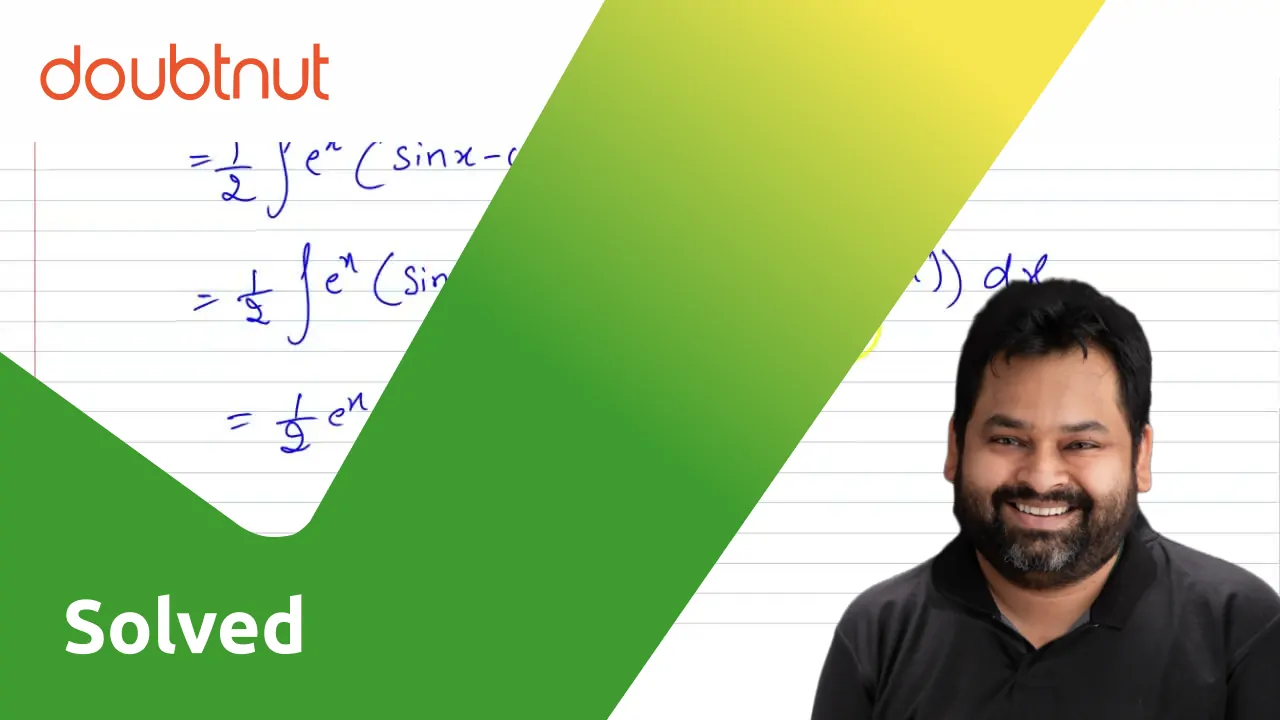Doubtnut App - Get 50 Lakh+ video solutions better than GoogleInstall Now

# Each question has four choices, a,b,c and d, out of which only one is correct. Each question contains STATEMENT 1 and STATEMENT 2. (a) If both the statements are TRUE and STATEMENT 2 is the correct explanation of STATEMENT 1. (b) If both the statements are TRUE but STATEMENT 2 is NOT the correct explanation of STATEMENT 1. (c) If STATEMENT 1 is TRUE and STATEMENT 2 is FALSE. (d) If STATEMENT 1 is FALSE and STATEMENT 2 is TRUE. Statement 1: ∫exsinxdx=ex2(sinx−cosx)+c Statement 2: ∫ex(f(x)+f′(x))dx=exf(x)+c

Video Solution